# logistic回归的应用

在上文中提到图片相似度比对算法，得出了图片的相似度数据。接下来最重要的是通过相似度数据来得出图片是否相似，也就是对于多个数据进行计算得到相似与不相似两个结果。这就要使用分类回归——logistic回归了。由于本人对于机器学习造诣不足，本文不过多的涉及回归模型的原理讲解，只对模型的java实现与模型在本项目中的应用进行阐述。

## Logistic回归的直观认识

线性回归模型想必大家都知道，它是对于数据的拟合，通过学习多组数据，得出一条可以很好拟合所有数据的方程（模型）。再将新数据带入这个方程（模型），就可以计算得出一个数值，即预测结果。当然这个方程是多元的，因为影响结果的因素不止一个，也不一定是一次的，因为变量之间的关系可能不是一维的。选择不同的方程，得出的预测准确度是不尽一样的。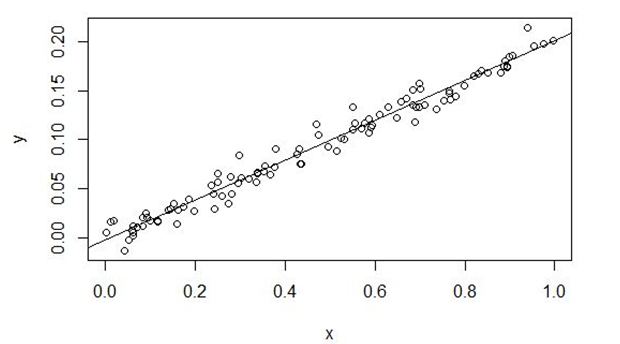（线性回归：一元一次方程得出的是一条直线）

Logistic分类回归可以理解为就是对线性回归得出结果的进一步计算，将结果限定在0和1之间，从而表示相似与不相似。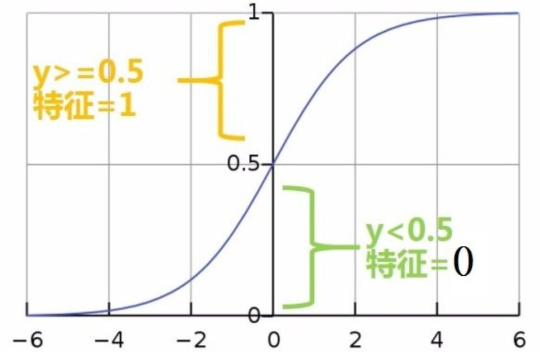（logistic函数：将结果限定在0与1之间）

当然整个重点就是在于怎么拟合训练数据，常用的算法就是梯度下降。通过梯度下降可以让我们的方程逐步的向最小误差靠近，这里逐步的步长是可以改变的，也就是学习率。学习率的选择是比较重要的，会影响整个模型的学习速度与正确率。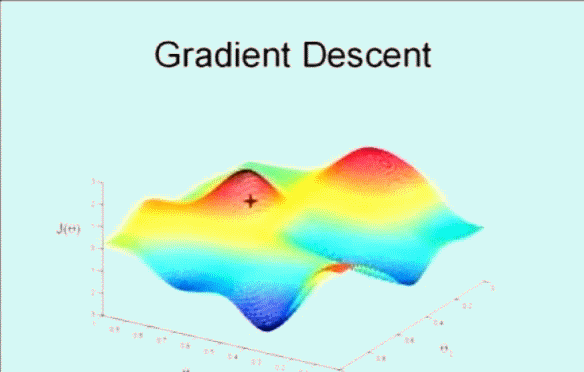（梯度下降：算法逐步靠近局部最优值）

总的来说，通过logistic模型，可以对我们输入的相似度数据计算出一个0到1的相似值，通过该值就可以确定图片是否相似，从而识别文字。

## 带入数据进行训练

训练的数据是两张图片的对比相似度，如果图片是同一个字，我们将结果标记为1，否则标记为0。下面是一些数据，x为多个相似度算法得出的结果，y为结果标记，为了方便观察，对数据进行了一些处理。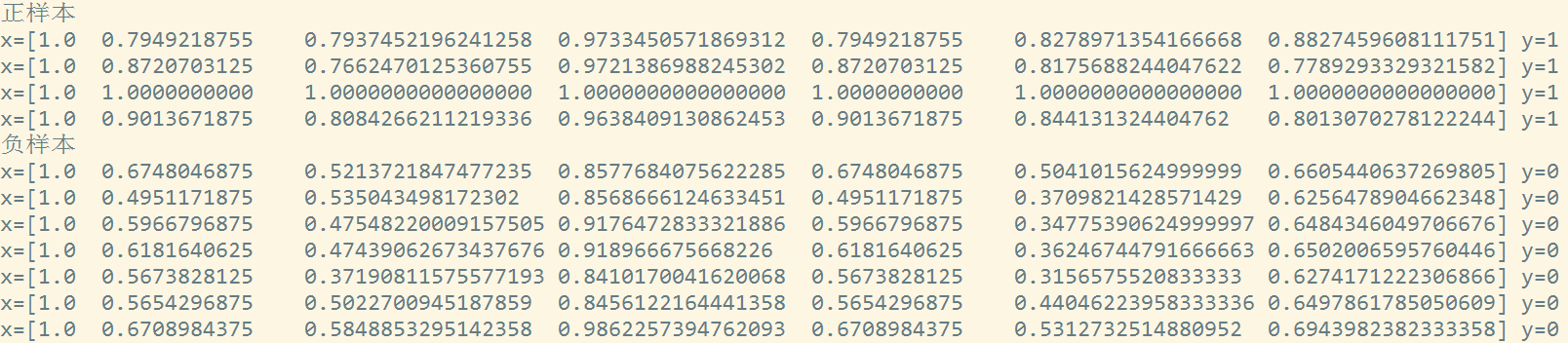可以看到x0一直为1，这是因为在方程中第一项是常数项，即x的0次方为1，也可以理解为偏置。还有6个对比算法得出相似度数据，可以看到正样本中的相似度比负样本中的高，说明我们的对比算法是有一定说服力的。但是每种算法的准确率不尽一样比如x3的正负样本都得出了比较高的相似度，说明准确率不高。因为x3是基于重心的对比，方块字的重心都差不多，所以没什么说服力。将这些数据带进模型进行训练，可以得出如下结果。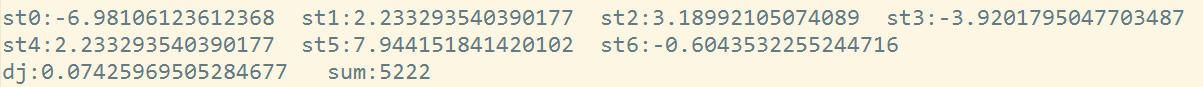可以看到经过5222次的迭代，结果和我们预测的差不多，x3和x6与结果呈负相关。整个代价0.074，即准确率92.6%，已经不错了，毕竟学习数据很少。

## 使用训练好的模型

上文我们已经将模型训练好了，现在只需要将相似度比对数据带入模型即可得出预测结果。结果是一个0~1之间的数据，我们将待预测图片与字库图片进行一一比对后带入模型，取结果最大的为识别结果即可。

## 模型的java实现

package util;

import java.util.List;

import java.util.Map;

/**

* @Description 分类回归模型，传入训练集，学习得到sts，即可使用sts对输入的x计算y

* @date 2018年7月16日 下午2:33:31

*/

public class LogisticModel {

private double[] sts=null;//参数Θ，通过学习得到

private double a=0.1;//学习速率

private List<Map<String,Object>> list=null;

/**

* @param list 训练集 Map中x为 double[]代表变量数组；y为double代表结果

*/

public LogisticModel(List<Map<String,Object>> list){

this.list=list;

Map<String,Object> map=list.get(0);//空指针异常，未判断

double[] x=(double[])map.get("x");//空指针异常，未判断

sts=new double[x.length];

}

public LogisticModel(){

}

/**

* @Description 函数模型 X1*Θ1+...+Xn*Θn=y。输入x计算y

* @param xs x变量

* @return 计算结果

* @date 2018年7月16日 下午2:25:10

*/

public double function(double[] xs){

double re=0f;

for(int i=0;i<xs.length;i++){//X1*Θ1+...+Xn*Θn=y 全是一维，对于本次学习足以

re+=xs[i]*sts[i];

}

return 1/(Math.pow(Math.E, -re)+1);//logistic函数，将结果限定在0~1

}

/**

* @Description 使用梯度下降算法进行函数参数更新（学习）

* @date 2018年7月16日 下午2:28:09

*/

private void update(){

double[] stss=new double[sts.length];//新的模型参数，此处单独用数组来装而不是直接对该参数赋值，是为了不影响下一个参数的学习，保证每个参数对应的都是同一个函数

int len=list.size();

for(int i=0;i<stss.length;i++){//遍历每一个参数

double sum=0f;

for(Map<String,Object> map:list){

double[] xs=(double[])map.get("x");

double y=(double)map.get("y");

sum+=(function(xs)-y)*xs[i];

}

//System.out.println("js---:"+a*(1.0f/len)*sum);

stss[i]=sts[i]-a*(1.0f/len)*sum;//更新该参数

}

sts=stss;//统一更新参数

}

/**

* @Description 代价函数，在训练集上计算误差

* @return 误差损失

* @date 2018年7月16日 下午2:29:36

*/

private double dj(){

double sum=0f;

for(Map<String,Object> map:list){

double[] xs=(double[])map.get("x");

double y=(double)map.get("y");

sum+=y*Math.log(function(xs))+(1-y)*Math.log(1-function(xs));

}

return -(1.0f/list.size())*sum;

}

/**

* @Description 开始学习

* @date 2018年7月16日 下午2:30:51

*/

public void go(){

int sum=0;//参数迭代次数

int count=0;

while(true){

double oldDj=dj();//迭代前损失

sum++;

if(sum>=10000){//迭代次数不超过10000

break;

}

update();//迭代

double newDj=dj();//迭代后损失

if(Math.abs(newDj-oldDj)<0.00001){//两次损失差

count++;

if(count>10){//如果损失差小于0.00001连续10次,则认为已经拟合

break;

}

}else{count=0;}

}

for(int j=0;j<sts.length;j++){

System.out.print("st"+j+":"+sts[j]+"  ");//输出学习到的所有参数

}

System.out.println("\ndj:"+dj()+"   sum:"+sum);//输出误差已经总学习次数

}

public double[] getSts() {

return sts;

}

public void setSts(double[] sts) {

this.sts = sts;

}

public List<Map<String, Object>> getList() {

return list;

}

public void setList(List<Map<String, Object>> list) {

this.list = list;

}

}

## 训练模型的代码

package test;

import java.io.File;

import java.io.IOException;

import java.util.ArrayList;

import java.util.HashMap;

import java.util.List;

import java.util.Map;

import imgdo.ImgData;

import util.ImgUtil;

import util.LogisticModel;

/**

* @Description

* @date 2018年8月3日 上午11:41:54

*/

public class LogisticTest {

private String trueFolder="F:\\textImg\\trueFolder";

private String falseFolder="F:\\textImg\\falseFolder";

public static void main(String[] args) throws IOException {

new LogisticTest().learn();

}

/*

* 下面这个方法只对一个字进行了学习。将同样的字作为正样本，其他字为负样本，进行分类学习

* 如果衍生到所有字：

* 将所有字放在一起，文件名为该字。学习时判断文件名是否一致，一致则结果设置为1.其他为0

* 当然集合中一致的字是相对少的，这样会导致负样本过多，可以随机取固定比例的负样本进行学习。

* */

public void learn() throws IOException{

ImgUtil iu=new ImgUtil();

File trueFile=new File(trueFolder);

File[] trueFiles=trueFile.listFiles();

File falseFile=new File(falseFolder);

File[] falseFiles=falseFile.listFiles();

List<Map<String,Object>> list=new ArrayList<Map<String,Object>>();

for(int i=0,len=trueFiles.length;i<len;i++){//将正样本进行两两比较

for(int j=i+1;j<len;j++){

ImgData data=iu.formatLibImg(trueFiles[i]),img=iu.formatLibImg(trueFiles[j]);//格式化图片

double[] re=iu.allMatch(data, img);//比对图片，得出各个比对算法的计算结果

Map<String,Object> map=new HashMap<String,Object>();

System.out.println("x=["+re+"\t"+re+"\t"+re+"\t"+re+"\t"+re+"\t"+re+"\t"+re+"]\ty=1");

map.put("x", re);

map.put("y",1.0);//结果为相似

}

}

for(int i=0,len=trueFiles.length;i<len;i++){//将正样本与每一个负样本进行比较

for(int j=0,jLen=falseFiles.length;j<jLen;j++){

ImgData data=iu.formatLibImg(trueFiles[i]),img=iu.formatLibImg(falseFiles[j]);//格式化图片

double[] re=iu.allMatch(data, img);//比对图片，得出各个比对算法的计算结果

Map<String,Object> map=new HashMap<String,Object>();

System.out.println("x=["+re+"\t"+re+"\t"+re+"\t"+re+"\t"+re+"\t"+re+"\t"+re+"]\ty=0");

map.put("x", re);

map.put("y",0.0);//结果为不相似

}

}

LogisticModel lm=new LogisticModel(list);//带入模型

lm.go();//开始学习

}

}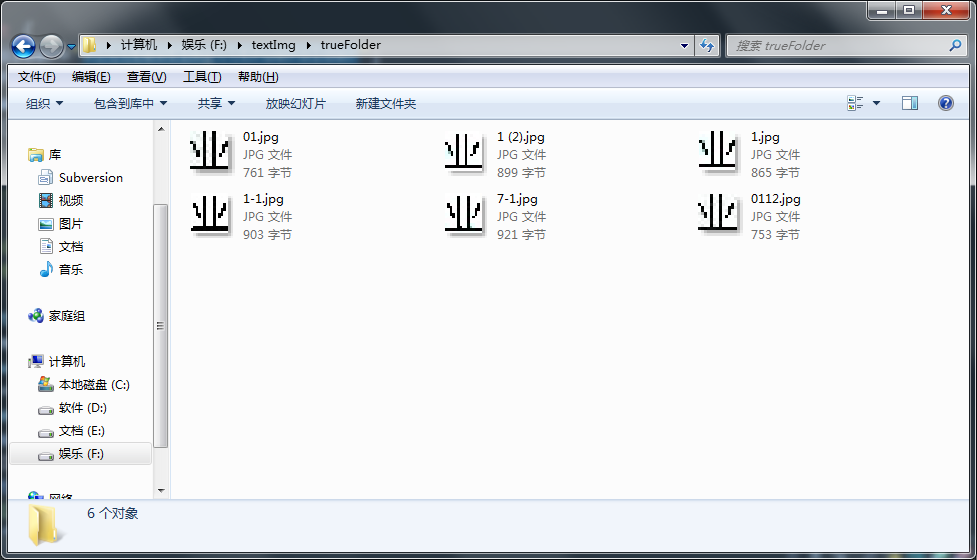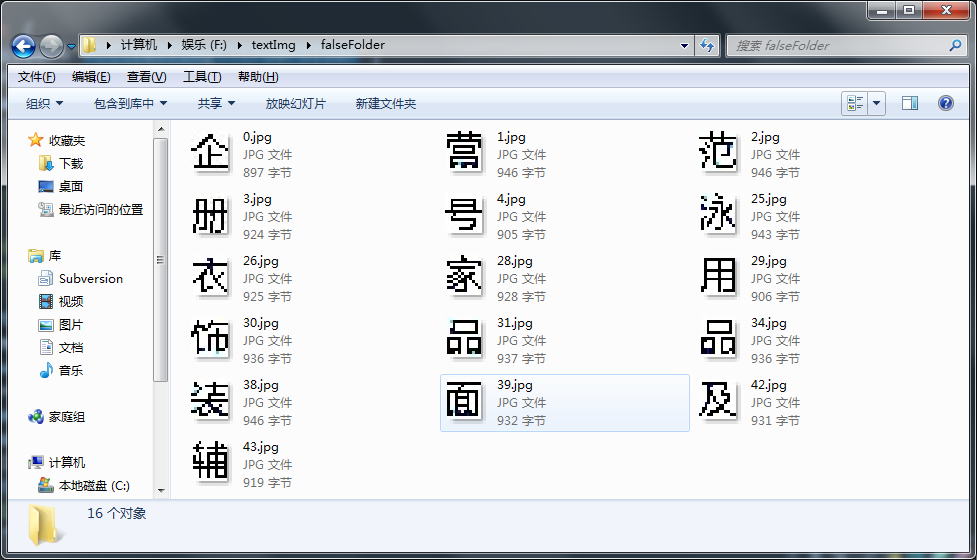02-28
12-22237

06-093703
04-132万+
02-133524
04-214553
05-091332
07-154万+
06-155万+
03-301369
02-10631
04-021608
09-283720
02-27332
10-281451
09-21703
02-173514
09-181468
08-311万+

### “相关推荐”对你有帮助么？

•非常没帮助
•没帮助
•一般
•有帮助
•非常有帮助Print_lin

¥2 ¥4 ¥6 ¥10 ¥20余额支付 (余额：-- )扫码支付获取中扫码支付点击重新获取扫码支付1.余额是钱包充值的虚拟货币，按照1:1的比例进行支付金额的抵扣。
2.余额无法直接购买下载，可以购买VIP、C币套餐、付费专栏及课程。余额充值